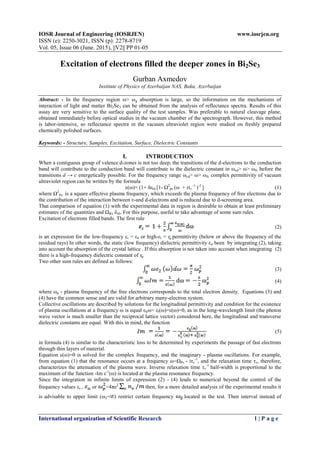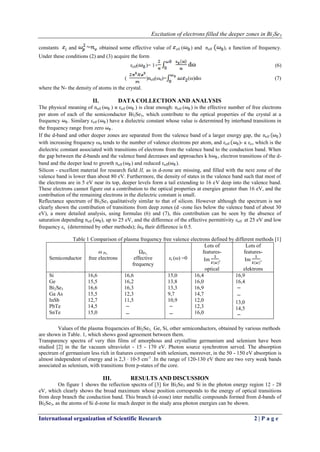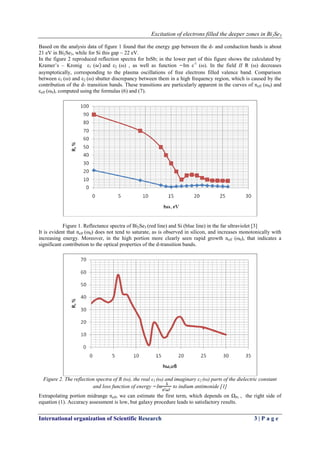Seu SlideShare está sendo baixado. ×
Anúncio
Anúncio
Anúncio
Anúncio
Anúncio
Anúncio
Anúncio
Anúncio
Anúncio
Anúncio
AnúncioAnúncio
Carregando em…3
×

1 de 5 Anúncio

# A05620105

IOSR Journal of Engineering Volume 5 Issue 6 Version 2

IOSR Journal of Engineering Volume 5 Issue 6 Version 2

Anúncio
Anúncio

### A05620105

1. 1. IOSR Journal of Engineering (IOSRJEN) www.iosrjen.org ISSN (e): 2250-3021, ISSN (p): 2278-8719 Vol. 05, Issue 06 (June. 2015), ||V2|| PP 01-05 International organization of Scientific Research 1 | P a g e Excitation of electrons filled the deeper zones in Bi2Se3 Gurban Axmedov Institute of Physics of Azerbaijan NAS, Baku, Azerbaijan Abstract: - In the frequency region ω> ωg absorption is large, so the information on the mechanisms of interaction of light and matter Bi2Se3 can be obtained from the analysis of reflectance spectra. Results of this assay are very sensitive to the surface quality of the test samples. Was preferable to natural cleavage plane, obtained immediately before optical studies in the vacuum chamber of the spectrograph. However, this method is labor-intensive, so reflectance spectra in the vacuum ultraviolet region were studied on freshly prepared chemically polished surfaces. Keywords: - Structure, Samples, Excitation, Surface, Dielectric Constants I. INTRODUCTION When a contiguous group of valence d-zones is not too deep, the transitions of the d-electrons to the conduction band will contribute to the conduction band will contribute to the dielectric constant in ωcd> ω> ωfʋ before the transitions d → c energetically possible. For the frequency range ωcd> ω> ωfʋ complex permittivity of vacuum ultraviolet region can be written by the formula ε(ω)= (1+ δε0) [1- Ω2 pʋ (ω + iτv -1 )-2 ] (1) where Ω2 Pʋ is a square effective plasma frequency, which exceeds the plasma frequency of free electrons due to the contribution of the interaction between ʋ-and d-electrons and is reduced due to d-screening area. That comparison of equation (1) with the experimental data in region is desirable to obtain at least preliminary estimates of the quantities and ΩRv δε0. For this purpose, useful to take advantage of some sum rules. Excitation of electrons filled bands. The first rule (2) is an expression for the low-frequency εi = εδ or high-εi = ε͚ permittivity (below or above the frequency of the residual rays) In other words, the static (low frequency) dielectric permittivity εδ been by integrating (2), taking into account the absorption of the crystal lattice . If this absorption is not taken into account when integrating (2) there is a high-frequency dielectric constant of ε͚. Two other sum rules are defined as follows: (3) (4) where ωp - plasma frequency of the free electrons corresponds to the total electron density. Equations (3) and (4) have the common sense and are valid for arbitrary many-electron system. Collective oscillations are described by solutions for the longitudinal permittivity and condition for the existence of plasma oscillations at a frequency ω is equal εl(ω= εl(ω)=ε(ω)=0, as in the long-wavelength limit (the photon wave vector is much smaller than the reciprocal lattice vector) considered here, the longitudinal and transverse dielectric constants are equal. With this in mind, the function (5) in formula (4) is similar to the characteristic loss to be determined by experiments the passage of fast electrons through thin layers of material. Equation ε(ω)=0 is solved for the complex frequency, and the imaginary - plasma oscillations. For example, from equation (1) that the resonance occurs at a frequency ω=ΩPv - iτv -1 , and the relaxation time τv, therefore, characterizes the attenuation of the plasma wave. Inverse relaxation time τv -1 half-width is proportional to the maximum of the function -Im ε-1 (ω) is located at the plasma resonance frequency. Since the integration in infinite limits of expression (2) - (4) leads to numerical beyond the control of the frequency values εs , or =4πe2 then, for a more detailed analysis of the experimental results it is advisable to upper limit (ω2= ) restrict certain frequency located in the test. Then interval instead of
2. 2. Excitation of electrons filled the deeper zones in Bi2Se3 International organization of Scientific Research 2 | P a g e constants and obtained some effective value of еff ( ) and neff ), a function of frequency. Under these conditions (2) and (3) acquire the form εeIf( )= 1+ (6) ( )neff(ω0)= (ω)dω (7) where the N- the density of atoms in the crystal. II. DATA COLLECTION AND ANALYSIS The physical meaning of neff ( ) и εeff ( ) is clear enough: neff ( ) is the effective number of free electrons per atom of each of the semiconductor Bi2Se3, which contribute to the optical properties of the crystal at a frequency . Similary εeff ( ) have a dielectric constant whose value is determined by interband transitions in the frequency range from zero . If the d-band and other deeper zones are separated from the valence band of a larger energy gap, the neff with increasing frequency ω0 tends to the number of valence electrons per atom, and εeff )- к εcv which is the dielectric constant associated with transitions of electrons from the valence band to the conduction band. When the gap between the d-bands and the valence band decreases and approaches k h , electron transitions of the d- band and the deeper lead to growth neff ( ) and reduced εeff( ). Silicon - excellent material for research field II, as in d-zone are missing, and filled with the next zone of the valence band is lower than about 80 eV. Furthermore, the density of states in the valence band such that most of the electrons are in 5 eV near its top, deeper levels form a tail extending to 16 eV deep into the valence band. These electrons cannot figure out a contribution to the optical properties at energies greater than 16 eV, and the contribution of the remaining electrons in the dielectric constant is small. Reflectance spectrum of Bi2Se3 qualitatively similar to that of silicon. However although the spectrum is not clearly shown the contribution of transitions from deep zones (d -zone lies below the valence band of about 30 eV), a more detailed analysis, using formulas (6) and (7), this contribution can be seen by the absence of saturation depending neff ), up to 25 eV, and the difference of the effective permittivity εeff at 25 eV and low frequency εs (determined by other methods); δε0 their difference is 0.5. Table 1 Comparison of plasma frequency free valence electrons defined by different methods  Semiconductor ω Pʋ, free electrons ΩРʋ, effective frequency εi (ω) =0 Lots of features- Im , optical Lots of features- Im , elektrons Si Ge Bi2Se3 Ga As InSb PbTe SnTe 16,6 15,5 16,6 15,5 12,7 14,5 15,0 16,6 16,2 16,3 12,3 11,5 15,0 13,8 13,3 9,7 10,9 16,4 16,0 16,9 14,7 12,0 12,3 16,0 16,9 16,4 13,0 14,5 Values of the plasma frequencies of Bi2Se3, Ge, Si, other semiconductors, obtained by various methods are shown in Table. 1, which shows good agreement between them. Transparency spectra of very thin films of amorphous and crystalline germanium and selenium have been studied  in the far vacuum ultraviolet - 15 - 170 eV. Photon source synchrotron served. The absorption spectrum of germanium less rich in features compared with selenium, moreover, in the 50 - 150 eV absorption is almost independent of energy and is 2,3 ∙ 10-5 cm-1 .In the range of 120-130 eV there are two very weak bands associated as selenium, with transitions from p-states of the core. III. RESULTS AND DISCUSSION On figure 1 shows the reflection spectra of  for Bi2Se3 and Si in the photon energy region 12 - 28 eV, which clearly shows the broad maximum whose position corresponds to the energy of optical transitions from deep branch the conduction band. This branch (d-zone) inter metallic compounds formed from d-bands of Bi2Se3, as the atoms of Si d-zone lie much deeper in the study area photon energies can be shown.
3. 3. Excitation of electrons filled the deeper zones in Bi2Se3 International organization of Scientific Research 3 | P a g e Based on the analysis data of figure 1 found that the energy gap between the d- and conduction bands is about 21 eV in Bi2Se3, while for Si this gap ~ 22 eV. In the figure 2 reproduced reflection spectra for InSb; in the lower part of this figure shows the calculated by Kramer’s – Kronig ε1 ( and ε2 (ω) , as well as function Im ε-1 (ω). In the field II R (ω) decreases asymptotically, corresponding to the plasma oscillations of free electrons filled valence band. Comparison between ε1 (ω) and ε2 (ω) shutter discrepancy between them in a high frequency region, which is caused by the contribution of the d- transition bands. These transitions are particularly apparent in the curves of neff (ω0) and εeff (ω0), computed using the formulas (6) and (7). Figure 1. Reflectance spectra of Bi2Se3 (red line) and Si (blue line) in the far ultraviolet  It is evident that nеff (ω0) does not tend to saturate, as is observed in silicon, and increases monotonically with increasing energy. Moreover, in the high portion more clearly seen rapid growth neff (ω0), that indicates a significant contribution to the optical properties of the d-transition bands. Figure 2. The reflection spectra of R (ω), the real ε1 (ω) and imaginary ε2 (ω) parts of the dielectric constant and loss function of energy Im to indium antimonide  Extrapolating portion midrange neff, we can estimate the first term, which depends on ΩРʋ , the right side of equation (1). Accuracy assessment is low, but galaxy procedure leads to satisfactory results.
4. 4. Excitation of electrons filled the deeper zones in Bi2Se3 International organization of Scientific Research 4 | P a g e All connections Bi2Se3 in the saturation region ( eV ) ε eff much less low-frequency permittivity εs, determined by independent methods, the value of δ 0 = 0.3 - 0.8 . Comparing the energy corresponding to the maximum function with values obtained by other methods, carried out in table 1 Bi2Se3. Here agreement less convincing than in the case of silicon, but quite satisfactory. In  studied the optical properties of a number of А3В5 compounds at 300 0 К in the 15 -170 eV. Quanta of radiation from a synchrotron is passed through a thin amorphous and crystalline layers of InSb, GaSb, AlSb, GaAs, GaP . The spectra Bi2Se3 are similar to the spectrum of InSb, and GaAs spectrum similar in structure spectra of GaP and Ge. In the area of up to 40 eV narrow bands are due to transitions from the d-conduction band and absorption structure roughly reproduces the structure of the density of states in the conduction band. A broad band of continuous absorption in the region 40-130 eV typical for all and compounds containing elements of the fifth and more low - rows of the periodic table. Structure comprising a narrow p-bands in the spectra of these compounds does not occur. Continuous absorption at 40 - 150 eV, for Bi2Se3, much weaker for compounds containing the third and fourth row of the periodic table, but in this area visible narrow band due to transitions from p - levels of the ion core. Based on the analysis of the asymptotic part of the absorption spectrum, using the formula (3) for plasma lap determine the relaxation time ʋ= h , when Еʋ=5,2 eV, т. е. ʋ = 1,3 -16 s. When calculating the number of electrons per atom was assumed equal to five (nʋ=5) . On the figure 2 shows the experimental and calculated dependences ε1 (ω) and ε2 (ω) in 10-20 eV for SnTe. This is quite satisfactory agreement with the experimental dependences. Comparison of peak energy function -Imε-1 (ω) and energy-loss obtained from experiments on the passage of electrons through the film does not give good agreement, apparently, due to the imperfections of the films used. However, for example, for PbTe these energy-loss EL and plasma resonance EР coincide (EL=ЕР= 14,5 eV). Height reflection spectrum Bi2Se3 and other lead chalcogenides at high energies (above 15 eV) can be attributed to transitions of electrons from the filled d-band to the conduction band, since the d-band in lead chalcogenides formed from d-bands of lead atoms and is located below conduction band 24 eV. In Bi2Se3 d-zone is much deeper and is therefore in the reflection spectrum in the energy range up to 20 eV does not occur. The growth trend reflected at energies more than 20 eV prompted to perform research  transparency PbTe and PbS films of thickness 1000 A at higher energies. Films were prepared on a thin carbon substrate, coated on a copper grid, a source of photons with energy of transparency and not reflections (less than 1%) in this energy region. Figure 3 shows spectra of the absorption coefficient for Bi2Se3 and Si, in which there is no fine structure, but has a broad maximum at 82 eV for Bi2Se3 and 58 eV for Si [5-9]. These peaks are attributed to transitions d within the same electron shell. Figure 3. The absorption spectra of α (E) and the effective electron density Nеff Si (blue line)and Bi2Se3 (red line) in the vacuum ultraviolet region
5. 5. Excitation of electrons filled the deeper zones in Bi2Se3 International organization of Scientific Research 5 | P a g e IV. CONCLUSION The spectra of the absorption coefficient α (E) were analyzed by the formula Neff 15 3 (8) where Neff is the effective density of states, the oscillator strength which is depleted in the region between the energies E1 and E2, the electron / molecule; α-lattice constant; n(E) is a real part of the refractive index. The calculation of the imaginary part of the refractive index (absorption index k (E)) on the basis of the absorption coefficient α (E) shows that n (E) differs from the unit for not more than 10% (the maximum value of n (E) similar to the maximum k (E) for the observed line width). Because of this, and taking into account a qualitative sense Nеff in solving (8) on the basis of the experimental spectrum α (E) believed that n (E) = 1. The value Nеff calculated with E1 = 37 eV, shown in figure 3 depending on the E2. Can be seen that at 150 eV Nеff, when the oscillator strength is exhausted, was 10.6 to Bi2Se3 and 22.5 for Si. It is assumed that in the case of lead sulfide includes Neff all the 5d-electrons of the atoms of lead, and in the case of lead telluride to 5d- electrons of the atoms of lead, and in the case of lead telluride to 5d-electrons of the atoms of lead added 4d- electrons of the atoms of tellurium. REFERENCES  H. Philipp, H. Ehrenreich. Optical Properties of Semiconductors, Phys. Rev. 129, 1550, (1963)  M. Cardona et al. Optical Properties of Semiconductors from 12 to 200 ev, Proc. Intern. Conf. on the Phys. Of Semiconductors, Boston, 1970, p. 209.  H. Ehrenreich et al., Interband Transition in Group IV, III-V, and II-VI Semiconductors, Phys. Rev. Lett. 8, 59, (1962)  M. Cardona, R. Heasen. Optical Properties of Some Compound Semiconductors in the 36 - 150 ev, Region, Phys. Rev. B1, 2605 (1970).  G. Axmedov. Physical Properties and Influence of the Tempering on Electric Properties Films Bi2Te3, International Journal of Materials Science and Applications. Vol. 3, No. 3, 2014, pp. 111-115. doi: 10.11648/j.ijmsa.20140303.17  G. Axmedov. Phase formation in bite nanofilms and crystallization of Bi2Te3 (Se3) nanothickness amorphous film. Scientifik Israel – technological advantages, Vol.14, 2012, No.4 s.85-94  D. Ismailov., G. Axmedov, R. Shafizade // Reports of Academy of Azerbaijan (1989). vol. XLV. no.4. pp. 4. (in Russian)  Physical properties of the films Вi2Te3-Bi2Se3 and thermophotovoltaic elements on their basis. American Journal of Physics and Applications 2014; 2(3): 83-87. Published online June 20, 2014 (http://www.sciencepublishinggroup.com/j/ajpa). doi: 10.11648/j.ajpa.20140203.13  N.Safarov, Axmedov G. , Axmedov S. Investigations of mathematical models in solar collectors American Journal of Energy Engineering 2014; 2(3): 75-79 Published online April 30, 2014 (http://www.sciencepublishinggroup.com/j/ajee) doi: 10.11648/j.ajee.20140203.11